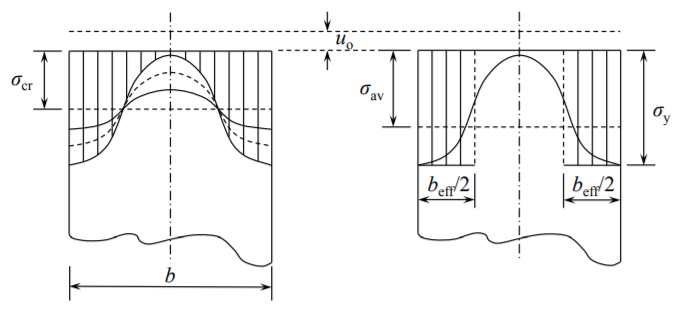# 10.6: Ultimate Strength of Plates

$$\newcommand{\vecs}{\overset { \rightharpoonup} {\mathbf{#1}} }$$ $$\newcommand{\vecd}{\overset{-\!-\!\rightharpoonup}{\vphantom{a}\smash {#1}}}$$$$\newcommand{\id}{\mathrm{id}}$$ $$\newcommand{\Span}{\mathrm{span}}$$ $$\newcommand{\kernel}{\mathrm{null}\,}$$ $$\newcommand{\range}{\mathrm{range}\,}$$ $$\newcommand{\RealPart}{\mathrm{Re}}$$ $$\newcommand{\ImaginaryPart}{\mathrm{Im}}$$ $$\newcommand{\Argument}{\mathrm{Arg}}$$ $$\newcommand{\norm}{\| #1 \|}$$ $$\newcommand{\inner}{\langle #1, #2 \rangle}$$ $$\newcommand{\Span}{\mathrm{span}}$$ $$\newcommand{\id}{\mathrm{id}}$$ $$\newcommand{\Span}{\mathrm{span}}$$ $$\newcommand{\kernel}{\mathrm{null}\,}$$ $$\newcommand{\range}{\mathrm{range}\,}$$ $$\newcommand{\RealPart}{\mathrm{Re}}$$ $$\newcommand{\ImaginaryPart}{\mathrm{Im}}$$ $$\newcommand{\Argument}{\mathrm{Arg}}$$ $$\newcommand{\norm}{\| #1 \|}$$ $$\newcommand{\inner}{\langle #1, #2 \rangle}$$ $$\newcommand{\Span}{\mathrm{span}}$$$$\newcommand{\AA}{\unicode[.8,0]{x212B}}$$

In the previous section we have shown that after buckling the plate continues to take additional load but with half of its pre-buckling stiffness. In order to understand what happens next, let’s examine the distribution of in-plane compressive stresses $$\sigma_{xx}$$ at $$x = a$$. From Equations (10.2.10-10.2.11) and (??) the components $$\sigma_{xx}$$ is

$\sigma_{xx}(y) = \frac{N_{xx}}{h} = \frac{E}{1 − \nu^2} \left[ −(1 − \nu^2) \frac{u_o}{a} + \frac{\pi^2}{2} \left(\frac{w_o}{a} \right)^2 \sin^2 \frac{\pi y}{a} \right]$

The first term represents negative, compressive stress, uniform along the width of the plate. The second term describes the relieving tensile stress produced by finite rotation. The relation between $$w_o$$ and $$u_o$$ is given by Equation (??) and is depicted in Figure (10.5.2). A plot of the function $$\sigma_{xx}(y)$$ for several values of the time-like parameter $$u_o$$ is shown in Figure ($$\PageIndex{1}$$). Note that the curves labeled A, B, C and D corresponds to the respective points in Figs. (10.5.2) and (10.5.3).Figure $$\PageIndex{1}$$: Re-distribution of compressive stresses along the loaded edge and simple approximation by von Karman.

With increasing plate compression there is a re-distribution of stresses along the loaded edge $$x = 0$$ and $$x = a$$. The stress at the unloaded edge $$y = 0$$ and $$y = a$$ keeps increasing while the stress at the plate symmetry plane $$y = \frac{a}{2}$$ diminishes to zero.

It was the German scientist and engineer, Theodore von Karman who in 1932 made use of the observation presented in Figure ($$\PageIndex{1}$$). He assumed that the central, unloaded portion of the plate carries zero stress while the edge zone, each of the width $$b_{\text{eff}}/2$$ reaches the yield stress at the point of ultimate load. As a starting point, von Karman used the expression for the critical buckling load $$N_c$$ and looked at the relation between the stress at the loaded edge $$\sigma_e$$ and the plate width $$b$$

$\sigma_e = \frac{N_e}{h} = \frac{N_c}{h} = \frac{4\pi^2D}{hb^2} = \frac{4\pi^2Eh^2}{12(1 − \nu^2)b^2} = 1.9^2E\left(\frac{h}{b}\right)^2$

Normally $$b$$ is the input parameter and the stress $$\sigma_e$$ is an unknown quantity. The ingenuity of von Karman was that he inverted what is known and unknown in Equation (??). He asked what should be the width of the plate $$b_{\text{eff}}$$ so that the edge stress reaches the yield stress. Thus

$\sigma_y = 1.9^2E\left(\frac{h}{b_{\text{eff}}}\right)^2$

Solving the above equation for $$b_{\text{eff}}$$

$b_{\text{eff}} = 1.9h \sqrt{\frac{E}{\sigma_y}}$

Taking for example $$E = 200000$$ MPa, $$b_{\text{eff}}\sigma_y = 320$$ MPa, the effective width becomes

$b_{\text{eff}} = 1.9h \sqrt{625} = 47.5h$

The effective width depends on the Young’s modulus and yield stress is proportional to the plate thickness. Approximately 40-50 thicknesses of the plate near the edges carries the load, the remaining central part is not effective. The total load on the plate can be expressed in two ways

$P_{\text{ult}} = b_{\text{eff}} \cdot \sigma_y = b \cdot \sigma_{\text{av}}$

where $$\sigma_{\text{av}} = \sigma_{\text{ult}}$$ is the average stress on the loaded edge at the point of ultimate strength,

$\frac{\sigma_{\text{av}}}{\sigma_{\text{ult}}} = \frac{b_{\text{eff}}}{b} = 1.9 \frac{h}{b} \sqrt{\frac{E}{\sigma_y}}$

The group of parameters

$\beta = \frac{b}{h} \sqrt{\frac{\sigma_y}{E}}$

is referred to as the slenderness ratio of the plate. Note that this is a different concept than the slenderness ratio of the column $$l/\rho$$. Using the parameter $$\beta$$, the ultimate strength of the plate normalized by the yield stress is

$\frac{\sigma_{\text{ult}}}{\sigma_{y}} = \frac{1.9}{\beta}$

Recall that the normalized buckling stress of the elastic plate is

$\frac{\sigma_{\text{cr}}}{\sigma_{y}} = \left(\frac{1.9}{\beta}\right)^2$

Plots of both functions are shown in Figure ($$\PageIndex{2}$$).

From this figure one can identify the critical slenderness ratio

$\beta_{\text{cr}} = 1.9$

when both the ultimate load and the critical buckling load reach yield. From Equation (??) one can see that at $$\beta = \beta_{\text{cr}}$$, the effective width is equal to the plate width, $$b_{\text{eff}} = b$$.Figure $$\PageIndex{2}$$: Dependence of the buckling stress and ultimate stress on the slenderness ratio.

Eliminating the parameter $$\beta$$ between Equations (??) and (??), the ultimate stress is seen to be the geometrical average between the yield stress and critical buckling stress

$\sigma_{\text{ult}} = \sqrt{\sigma_{\text{cr}} \cdot \sigma_{y}}$

For example, continuous loading of a plate with the slenderness ratio $$\beta_1$$ will first encounter the buckling curve and then the ultimate strength curve, as illustrated in Figure ($$\PageIndex{2}$$). The foregoing analysis was valid for plates simply supported along all four edges, for which the buckling coefficient is $$k_c = 4$$. For other type of support Equation (??) is still valid with the coefficient 1.9 replaced by 1.9 $$\frac{k_c}{4}$$.

Much effort has been devoted in the past to validate experimentally the prediction of the von Karman effective width theory. It was found that a small correction to Equation (??) provides good fit of most of the test data

$\frac{\sigma_{\text{ult}}}{\sigma_{y}} = \frac{b_{\text{eff}}}{b} = \frac{1.9}{\beta} − \frac{0.9}{\beta^2}$

For example, for a relatively short (stocky plate) $$\beta = 2\beta_{\text{cr}} = 3.8$$, the original formula over predicts by 15% than the more exact empirical equation (??). For slender plates, the difference is small. The latter has been the basis for the design of thin-walled compressive elements in most domestic and international standards such as AISI, Aluminum Association and AISC.

This page titled 10.6: Ultimate Strength of Plates is shared under a CC BY-NC-SA 4.0 license and was authored, remixed, and/or curated by Tomasz Wierzbicki (MIT OpenCourseWare) via source content that was edited to the style and standards of the LibreTexts platform; a detailed edit history is available upon request.